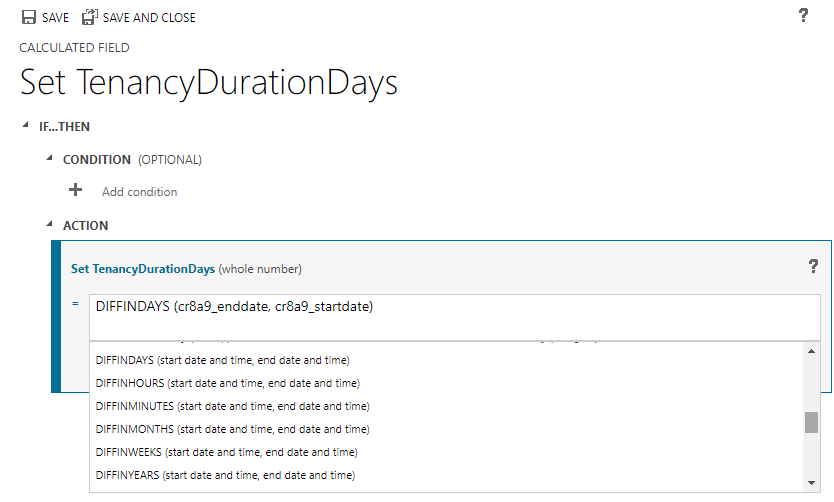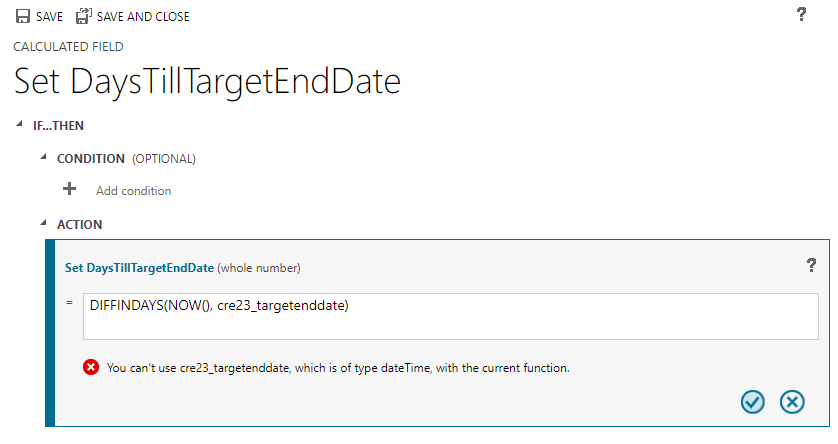# Dataverse - How to calculate durations with calculated columns

We can use calculated columns to calculate the difference between two date/time values. The available functions that apply to date fields work only between columns of the same behaviour type. This can cause problems, mostly in cases where we need to calculate an elapsed duration with the help of the Now() function. This post examines this issue, and offers a quick overview on how to define a calculated column.

We can determine the difference between two dates by using a calculated column. This method applies especially to model driven apps, where there is not the ability to define this type of logic at a screen/form level. There are many use case scenarios that require this type of calculation, and the typical ones may include:

• How to calculate the duration between the start and end dates of an activity
• How to calculate the number of days remaining until we reach a target end date
• How to calculate the number of days that of have elapsed since a start date
In this post, we'll walk through how to define a calculated column in Dataverse. A main focus of this post relates to a problem that app builders sometimes face when attempting to define a calculated column that calls the Now() function to calculate an elapsed time. We'll examine this issue in closer detail.

## Walkthrough - to define a calculated column

To demonstrate how to to define a calculated column, let's take the following table of tenancy details. Each tenancy record contains a start and end date value. Let's imagine that we want to create a calculated column that returns the number o days between these two values.To define a calculated column that returns this duration in days, we open the table in design view, add a new column, and click the Add > Calcution menu item.

##This opens a dialog (shown beneath) where we can define a formula for our calculated column.The functions that relate to date calculations include the following:

```ADDHOURS, ADDDAYS, ADDWEEKS, ADDMONTHS, ADDYEARS, SUBTRACTHOURS,
SUBTRACTDAYS, SUBTRACTWEEKS, SUBTRACTMONTHS, SUBTRACTYEARS, DIFFINDAYS,
DIFFINHOURS, DIFFINMINUTES, DIFFINMONTHS, DIFFINWEEKS, DIFFINYEARS```
The function names intuitively describe the purpose of each function, and the IntelliSense in the designer clarifies the arguments that each function expects. The online documentation provides full usage instructions for each of these functions.

At this stage, we can save our changes and the definition of our calculated column will be complete.

## Typical problem area - calling the Now() function in a calculated column

Problems usally occur in cases where we want define a calculated column that applies the Now() function. This is the function that returns the current date/time.

To demonstrate a typical error, let's take the example of a 'Project' table. This table includes a date column called 'TargetEndDate'. Our aim is to define a calculated column that returns the number of days between now and the 'TargetEndDate'.

If we attempt to define this calculated column, we may receive an error that says "you can't use 'ourDdatefield', which is of type datetime, with the current function" or alternatively, "you can't use Now(), which is of type datetime, with the current function".The reason this error occurs is because functions that calculate date differences work only with date columns of identical behaviour type.

With date fields, there are three behaviour types "User local", "Date only" and "Time zone independent". The most important difference between these types is that the "User local" behaviour type stores a time zone aware point in time. In contrast, the "Date only" behaviour type does not store any time zone information.

The Now() function works against source columns that are time zone aware. Therefore, it does not work against columns that are of behaviour type "Date only", and this is the typical reason why this type of error occurs. In this scenario, the fix is to change the behaviour type of the source column to "User local".## Conclusion

With Dataverse, there are a range of functions that apply to date calculations. These work only between date columns that share the same behaviour type. If we want to utilise the Now() function, we can use this only in conjunction with date fields that are of behaviour type "User local".